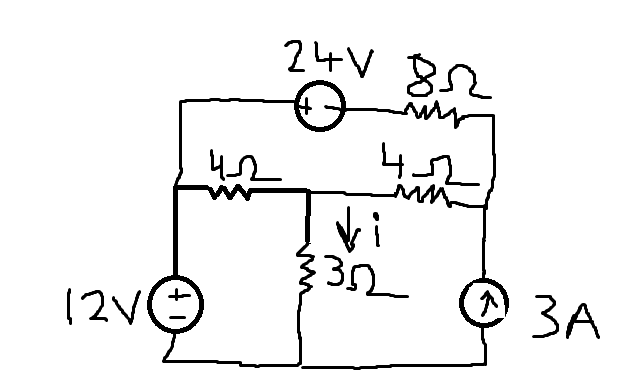# Finding the current through a resistor using superposition theorem.

Pottymouth

## Homework Statement

We have this circuit.The problem states to find i, or the current through the 3 ohm resistor.

V = IR

## The Attempt at a Solution

I've started by shorting out both voltage sources and leaving only the current source in the circuit. I paralleled the 4ohm resistor and the 3ohm resistor nearest the current source to get 12/7 ohms equivalent resistance. Multiplying that by 3A gives me 5.1 volts going through the 4 ohm resistor nearest the current source and 5.1 volts going through the 3 ohm resistor.

Dividing 5.1 volts by 3ohms gives me 1.7A going through the 3 ohm resistor from the current source alone. I'm not sure if did that right and need some help on what to do.

Gold Member
2022 Award
I've started by shorting out both voltage sources and leaving only the current source in the circuit.

So how do you reckon the current will be the same with and without the voltage sources? That is, you have been asked to find the current WITH the voltage sources in the circuit but you have solved for the current having replaced the voltage sources with wires. How do you figure you'll get the same answer?

Pottymouth
So how do you reckon the current will be the same with and without the voltage sources? That is, you have been asked to find the current WITH the voltage sources in the circuit but you have solved for the current having replaced the voltage sources with wires. How do you figure you'll get the same answer?

Ahh I should have clarified that I was going to solve it again with the other sources still there while the current source becomes an open circuit. I guess my real question was if I got the right amount of current through the 3 ohm resistor using the current source alone, which that will be added to the other currents I get when using the separate voltage sources alone.